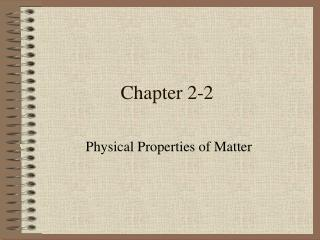DownloadDownload PresentationChapter 2-2

# Chapter 2-2

Télécharger la présentation## Chapter 2-2

- - - - - - - - - - - - - - - - - - - - - - - - - - - E N D - - - - - - - - - - - - - - - - - - - - - - - - - - -
##### Presentation Transcript

1. Chapter 2-2 Physical Properties of Matter

2. Physical Properties • A physical property of matter can be observed or measured without changing the matter’s identity. • Some examples of physical properties are state (phase), density, solubility, ductility, malleability, magnetism, and ability to conduct electricity.

3. Physical Properties • State (or phase) is the physical form in which a substance exists. • The states of matter are solid, liquid, gas, or plasma. • Density is the mass per unit volume of a substance. • Solubility is the ability of a substance to dissolve in another substance.

4. Physical Properties • Ductility is the ability of a substance to be pulled into wires. • Malleability is the ability of a substance to be rolled or pounded into thin sheets.

5. Ductility & Malleability

6. Density • Density is a physical property that describes the relationship between mass and volume. • Density is the amount of matter in a given space, or volume. • A golf ball and table-tennis (ping-pong) ball have similar volumes but the golf ball is denser because it has a greater mass.

7. Density • In Figure 4, page 46, why do you think maple syrup is on the bottom of the six liquids? Why do you think corn oil is on the top? • The denser liquids sink to the bottom of the flask. The less dense liquids rise to the top. • The order in which the layers separate shows the order of increasing density.

8. Density

9. Density • Knowing the density of a substance will tell you if the substance will float or sink in water. • The density of water (at 4°C) is 1.00 g/cm3. • If an object is less dense than water it will float in water. • If the object is more dense than water it will sink.

10. Density

11. Solving for Density • Density (D) is found by dividing mass (m) by volume (V). • Units for density consist of a mass unit divided by a volume unit. • Common units for density are g/cm3, g/mL, kg/m3, and kg/L.

12. Using Density to ID Substances • Each substance has a density that differs from the densities of other substances. • The density of a substance is always the same at a given temperature and pressure. • What is the density of Helium gas? • 0.00001663 g/cm3 • Write this in scientific notation. • 1.663 x 10-5 g/cm3

13. Using Density • What is the density of Mercury (the only liquid metal at room temperature)? • 13.55 g/cm3 • Read the Math Focus section of page 47. Try the three sample questions.

14. Using Density • What happens to the density of an object if the object is cut in half? Why? • The density remains the same because cutting the object in half will divide the mass & volume by the same amount. Also, the density of a substance remains the same no matter what size it is. All matter has its own unique density.

15. Interesting Density Fact • The density of a fresh egg is about 1.2 g/mL, and the density of a spoiled egg is about 0.9 g/mL. The density of the egg decreases as it ages because as it spoils, it loses water through the tiny pores in the shell. • What would happen to a fresh egg if placed in a beaker of water? What would happen to a spoiled egg?

16. Physical Changes • A physical change is a change that affects one or more physical properties of a substance. • Freezing water to make ice or sanding a piece of wood are examples of physical changes. • Physical changes do not change the identity of the matter involved, only some of the physical properties.

17. Phase Changes are Physical Changes